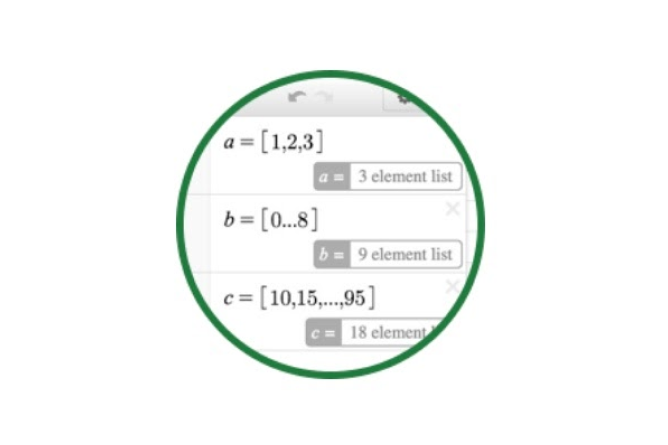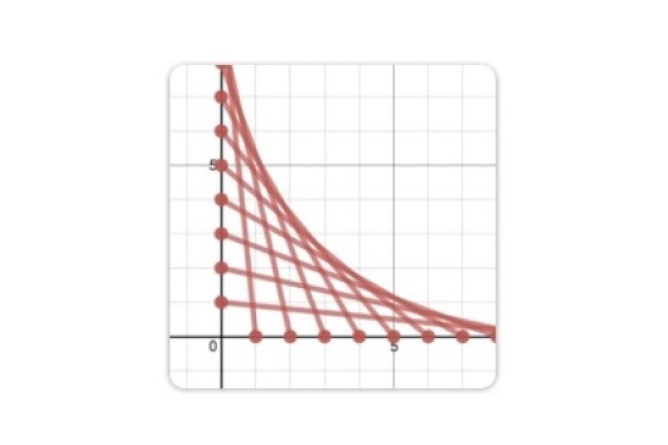# Lists

Define a list of values and calculate the mean, median, or standard deviation. Or use one or more lists to quickly plot a series of points, lines, or curves. Think of lists as the “two birds with one stone” feature in Desmos. Begin simply, and get as complex as you like. Get started with the video on the right, then dive deeper with the resources and challenges below.

You can make lists in Desmos using square brackets like this:You can create lists with evenly spaced elements. [1,...,10] is a list of the integers between 1 and 10. [1,3,...,11] is a list of the odd integers between 1 and 11. Table columns are available as lists in the rest of the system.

You can use lists anywhere in expressions that you would use a number.If L is a list, you can refer to the first element of L with L, produce a list of the first, third, and fifth elements of L with L[1,3,5], produce a list of the third through fifth elements of L with L[3...5], and produce a list of the third through last elements of L with L[3...]. If M is also a list, then L[M] produces a list of the elements of L given by the indices in M. You can also select the elements of a list that satisfy a condition: for example, if L is a list, then L[L>0] selects the positive elements of L, and L[mod(L,2)=0] selects the even elements of L.

#### List Comprehension

You can also create new lists based on existing lists using a list comprehension, which looks like this: $$\left(x,y\right)\operatorname{for}x=\left[1...10\right],y=\left[1...10\right]$$. This would create a list of the 100 points with integer coordinates $$1\le x \le 10$$, $$1\le y \le 10$$.

A list comprehension has a "body" expression--in this case (x,y)--and one or more "input list" variables. The resulting list comes from calculating the value of the body expression for each value of each input list variable. Here are some more examples of list comprehensions and the resulting lists they create:

Comprehension Result
$$2i\operatorname{for}i=\left[1,2,3\right]$$ $$[2, 4, 6]$$
$$1\operatorname{for}i=\left[1...1000\right]$$ $$[1, 1, 1, ...$$$$(1000$$ times)$$]$$
$$\operatorname{mean}\left(L.\operatorname{random}\left(10\right)\right)\operatorname{for}i=\left[1...100\right]$$ Take 100 different samples from list $$L$$ and compute the mean of each one.

## Lists in Action### Interactive Tour

Master the basics with a quick, step-by-step walkthrough.### Graphing Challenges